# Capacitor discharge through optocoupler

#### franck102

Joined Apr 7, 2020
5
Hi all,

I am trying to teach myself some basics to be less dependent on posted schematics... and not having much success so far.
I am trying to create a basic blinking led circuit using a relay, as described here: https://www.build-electronic-circuits.com/blinking-led-circuit/
I don't have a 5V relay so I am using one of those common 5V relay modules: http://www.techydiy.org/opto-isolated-dual-relay-module-arduino/

My module schematic looks like this: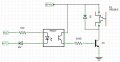I have a 1800 microF capacitor at hand, so I tried building an RC circuit like this (I have a separate 5V supply for the relay coil):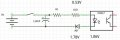The voltage drops (1.06V and 1.78V across the two diodes) are what I measured with the largest R1 that still closes the relay. In that setup R1 = 3.2kohm and If = 0.5mA

- estimate how long at most that capacitor can keep the relay closed
- find out what resistor will maximize that time

My problem is that I am not too sure how to reason about that circuit... here is what I came up with:
- I assume the tensions across the diodes will need to be at least the forward voltages I have measured above, 1.78V and 1.06V
- I assume the current If will need to be at least 0.5mA

... so I am using the equation for an RC discharge:
t = (R1 + 1kohm) * C * Ln( Vmin / V5)
where Vmin = 1.78V + 1.06V + (R1 + 1kohm) * 0.5mA

If I put the above in a spreadsheet I find a maximum t of about 0.95s for R1=1kohms; unfortunately experiene doesn't confirm that... when I disconnect Vcc the relay shuts down immediately (though the Led does decay in about 1s).

So my question is, how do you reason about that circuit? And how do you predict what capacitor you need to hold the relay for say 5s?

Thanks!
Franck
 R1 R2 C RC If Vs1 Vs2 Vmin / 5 t_on 3000​ 1000​ 1800μF​ 7.2​ 0.5mA​ 1.06V​ 1.78V​ 0.968​ 0.23416698​ 2000​ 1000​ 1800μF​ 5.4​ 0.5mA​ 1.06V​ 1.78V​ 0.868​ 0.764443247​ 1000​ 1000​ 1800μF​ 3.6​ 0.5mA​ 1.06V​ 1.78V​ 0.768​ 0.950275965​ 500​ 1000​ 1800μF​ 2.7​ 0.5mA​ 1.06V​ 1.78V​ 0.718​ 0.894471417​ 0​ 1000​ 1800μF​ 1.8​ 0.5mA​ 1.06V​ 1.78V​ 0.668​ 0.72624079​

#### Tonyr1084

Joined Sep 24, 2015
5,524
For a blinking LED you'd need some sort of switching circuit. Couple issues I see - and I'm no expert on this stuff - you have a 1KΩ resistor in series with another resistor of value 3.2KΩ. You have an opto isolator with a Vf of about 1.06 V (just call it 1V. You also have what is probably a red LED with a Vf of about 1.75 V. If those numbers are correct (the forward voltages that is) then you are dropping about 2.75 volts. With a 5 volt supply you have a remaining voltage of 2.25 volts. You also stated your current is 0.5 mA (that's 0.0005 amps). That's way too low to be of any service. The total resistance is (3.2K + 1K) 4.2KΩ Voltage divided by resistance gives you the current. So 5V -2.75V = 2.25V. 2.25V ÷ 4200Ω = 0.00054A That is too low to be of any value to make the circuit work. The capacitor ? ? ? it's serving no purpose for what I see.

The opto by itself should require at least 6 mA, not 0.5 mA and can probably work well with 10 mA. I'd recommend recalculating for a 10 mA circuit (second drawing) to control whatever it is you're out to control with the relay.

Other things you need to specify are what frequency you want the LED to flash at and its duty cycle. (i.e. one flash every second, on duration of 1/2 second, off 1/2 second for example) How much current the relay coil draws.

If you want a blinking LED to control a "blinking" relay then you're going to need more circuitry. Best solution would be a 555 timer chip you can control the frequency and duty cycle pretty easily.

#### Tonyr1084

Joined Sep 24, 2015
5,524
- estimate how long at most that capacitor can keep the relay closed
- find out what resistor will maximize that time
After re-reading your post it looks like you want to press a button and charge up a capacitor. When the button is released the cap continues to hold the relay (via the opto) for a set period of time. The 555 still offers the best solution, as a 555 can be set up as a monostable (one time - non-retriggerable) vibrator. Meaning when you push the button the 555 starts a countdown till it shuts off. Whether you hold the button for one second or one minute - the timer will perform the same timing function from the moment the switch is pressed. The RC timing would be far easier.

#### franck102

Joined Apr 7, 2020
5
After re-reading your post it looks like you want to press a button and charge up a capacitor. When the button is released the cap continues to hold the relay (via the opto) for a set period of time. The 555 still offers the best solution, as a 555 can be set up as a monostable (one time - non-retriggerable) vibrator. Meaning when you push the button the 555 starts a countdown till it shuts off. Whether you hold the button for one second or one minute - the timer will perform the same timing function from the moment the switch is pressed. The RC timing would be far easier.
Thanks Tony,

I do understand there are simpler ways to do this, and I have 555s and CD4541 CMOS timers on order. While waiting for those I was trying to learn the basics...

The circuit I posted is indeed only the 1st step towards blinking (where I will use the NC/NO contacts of the relay to alternate between feeding the capacitor+optocoupler and lighting a LED).

The optocoupler does happen to work fine with 0.5mA - I trust you that this is outside the specs and I wouldn't use that in a real project, but the relay is definitely steadily on with that current.

Here is the complete circuit I have right now: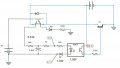Unfortunately the grounds of the 2 power supplies are connected (two rails of a breadboard supply), I'll try a separate coil supply next.
I do get a periodic relay switching, about 1s off / 0.5s on. The load LED sometimes lights up fine as expected, but most often it doesn't... so that circuit doesn't quite workI do hear the relay switching, so that part is working.

Franck

#### AlbertHall

Joined Jun 4, 2014
10,776
Note that the relay will need a good bit more more current to pull in than to maintain the pulled in state.

#### franck102

Joined Apr 7, 2020
5
Note that the relay will need a good bit more more current to pull in than to maintain the pulled in state.
Right, I went back to my original non-blinking version with just the relay module and 2 external resistors (+ 1k on the module) and at 0.6 mA the relay clicks and the test load LED turns on solidly, there is 0V across the relay contacts.
The capacitor starts at 4.7V (5V - VRc), but it doesn't hold the relay closed for any perceivable amount of of time.

The circuit is this: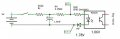When the switch is closed I measure 1.3V across R4 so unless I am completely off that's 0.6 mA.

In case this wasn't clear that's the current through the optocoupler's LED, the relay coil is powered separately from a separate power supply, from the module's transistor collector.

So... still wondering how to reason when I open the switch aboveI understand the current is probably borderline but I would still expect something around 0.75s given my calculations above.
Franck

#### Tonyr1084

Joined Sep 24, 2015
5,524
it doesn't hold the relay closed for any perceivable amount of of time.
You probably need a much much larger capacitor. 2200 µF 16V. Of course you'll have to adjust the resistance.

#### Alec_t

Joined Sep 17, 2013
11,797
I'm puzzled by your post #1 table. Vmin is a fixed value, so Vmin/5 should be too, and your t_on figures should vary monotonically with the RC time constant.

#### franck102

Joined Apr 7, 2020
5
I'm puzzled by your post #1 table. Vmin is a fixed value, so Vmin/5 should be too, and your t_on figures should vary monotonically with the RC time constant.
I used the formulas given in the post:
t = (R1 + 1kohm) * C * Ln( Vmin / V5)
where Vmin = 1.78V + 1.06V + (R1 + 1kohm) * 0.5mA

Vmin was the voltage cross the capacitor when the current is 0.5mA, so it does change with R1. And t varies with Ln(Vmin/V0).

This post explains why the approximation is not valid it seems:

https://electronics.stackexchange.c...citor-through-a-resistor-and-an-led-in-series

Franck

#### Alec_t

Joined Sep 17, 2013
11,797
I wrongly assumed Vmin was the voltage across the two LEDs in series.
Simulation suggests that with the total resistance varying between 1k and 4k you should be getting between 3.1 and 1.7 secs before the current drops below 0.5mA, so perhaps your 1800uF capacitor is ancient, leaky or wrongly marked?

#### franck102

Joined Apr 7, 2020
5
I wrongly assumed Vmin was the voltage across the two LEDs in series.
Simulation suggests that with the total resistance varying between 1k and 4k you should be getting between 3.1 and 1.7 secs before the current drops below 0.5mA, so perhaps your 1800uF capacitor is ancient, leaky or wrongly marked?
So my fundamental error was to assume that the RC discharge was to 0V, when it is in fact to Vd assuming that circuit: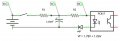This simulation shows that the current through the diodes drops down below 0.5A in about 1.1s: falstad
If the switch is opened at t=0 we have:
Code:
Vc(0) = Vd + R2/(R1+2) * (Vcc - Vd)

V(t) - Vd = (Vc(0) - Vd) * exp(-1/R2*C)
= R2/(R1+R2) * (Vcc-Vd) * exp(-1/R2*C)
Using a constant Vd = Vf = 2.84V as an approximation this yields the current If through the optocoupler:

Code:
If(t) = 1/R2 * (V(t)-Vd)
= 1/(R1+R2)(Vcc-Vd) * exp(-1/R2*C)
and so
t = -R2*C * Ln[ If(t) * (R1+R2) / (Vcc-Vd) ]
With R1 = 510ohms, R2 = 3.5k and If = 0.5mA I get 0.94s: not too bad, given that the simulator uses a variable forward voltage for the LEDsAs you mention the fact that the real circuit doesn't work is probably due to leakage current, series resistance of the diodes, and similar factors.

Franck